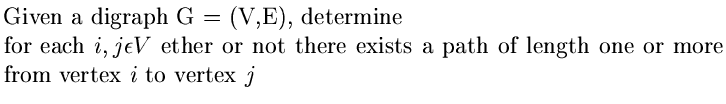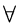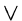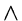Next:7.4 Depth First Search and Breadth First SearchUp:7. Directed GraphsPrevious:7.2.3 All Pairs Shortest Paths Problem: Floyd's Algorithm

# 7.3 Warshall's Algorithm

REF.
Stephen Warshall. A theorem on boolean matrices. Journal of the ACM, Volume 9, Number 1, pp. 11-12, 1962.Def: Given the adjacency matrix C of any digraph C = (v,E), the matrix A is called the transitive closure of C ifi, jtex2html_image_mark>#tex2html_wrap_inline27764# V,

 A[i, j] = 1 if there is a path of length one or more from vertex i to vertex j = 0 otherwise
Warshall's algorithm enables to compute the transitive closure of the adjacency matrix f any digraph. Warshall's algorithm predates Floyd's algorithm and simple uses the following formula in the kth passes of Floyd's algorithm:
Ak[i, j] = Ak - 1[i, j](Ak - 1[i, k]Ak - 1[k, j])
where the matrix elements are treated as boolean values 0 or 1 and the symbolsanddenote logical or'' and logical and'' respectively. It is easy to see that Warshall's algorithm has a worst case complexity of O(n3) where n is the number of vertices of the graph.Next:7.4 Depth First Search and Breadth First SearchUp:7. Directed GraphsPrevious:7.2.3 All Pairs Shortest Paths Problem: Floyd's Algorithm
eEL,CSA_Dept,IISc,Bangalore